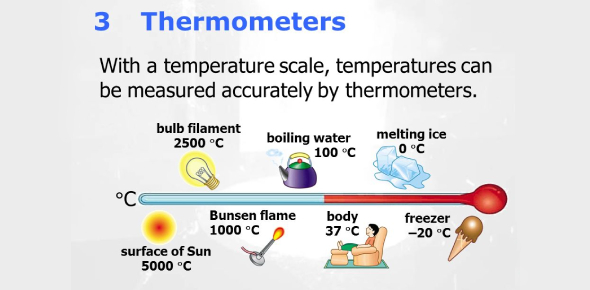# Temperature And Thermometer Questions! Trivia Quiz

15 Questions | Attempts: 8390SettingsAttempt these trivia quiz questions related to temperature and thermometer and check how updated you're about these two terms. Also, you'd be able to differentiate between temperature and thermometer here. Temperature tells how hot or cold something is, whereas a thermometer is an instrument used to measure the temperature of something. There are many such interesting things you'll get to know about in the following quiz. Shall we begin it now? Take up the test, then.

• 1.
We measure temperature in degrees _________.
• A.

Meter

• B.

Celsius

• C.

Fahrenheit

• D.

Both

• 2.
What is used to measure temperature?
• A.

A barometer

• B.

A thermometer

• C.

A gasometer

• D.

A seismometer

• 3.
The following is a type of thermometer except:
• A.

Digital thermometer

• B.

Graphic thermometer

• C.

Computerized thermometer

• D.

Visual Thermometer

• 4.
All of the following are temperature scales except:
• A.

Gabriel

• B.

Kelvin

• C.

Celsius

• D.

Fahrenheit

• 5.
Water freezes at:
• A.

100 degrees Celsius

• B.

0 degrees Celsius

• C.

37 degree Celsius

• D.

20°C

• 6.
Temperature is really a measure of how fast the atoms and molecules that make up a substance are moving.
• A.

True

• B.

False

• 7.
A digital thermometer shows the temperature on a scale or dial; an analog thermometer shows the temperature as a number.
• A.

True

• B.

False

• 8.
Kelvin is a measure of temperature that is abbreviated Kln.
• A.

True

• B.

False

• 9.
The degrees in Fahrenheit are smaller than those in Celsius.
• A.

True

• B.

False

• 10.
Water freezes at 0°C and boils at 100°C.
• A.

True

• B.

False

• 11.
Temperature is measured in many different scales, including __________, ___________ , and __________ scales.
• 12.
The fahrenheit scale was developed by_____________________.
• 13.
Normal human body temperature is ______________________.
• 14.
An analog thermometer shows the temperature on a__________________.
• 15.
A digital thermometer shows the temperature as a ___________________________.

## Related TopicsBack to top
×

Wait!
Here's an interesting quiz for you.